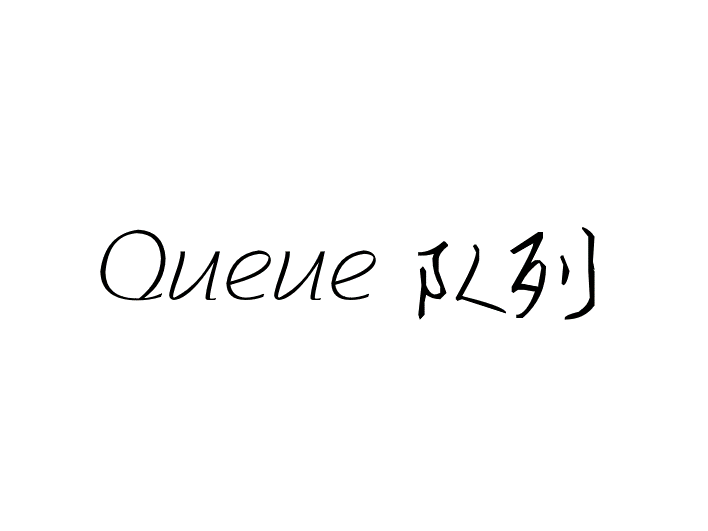Java底层实现Queue队列

# Queue 队列

Github LiYangSir
Home https://quguai.cn
Language Java

## 1、什么是队列

Stack -> 先进后出，后进先出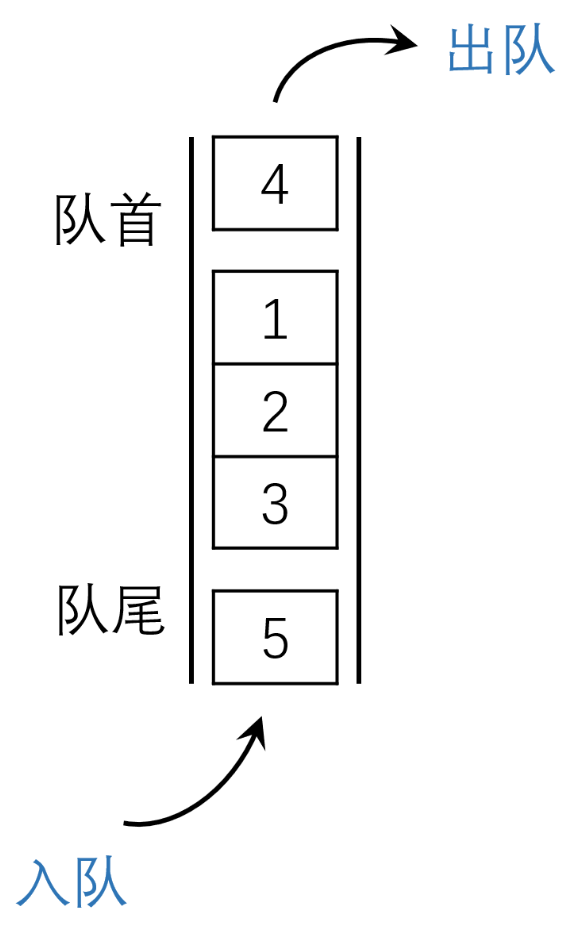public interface Queue<E> {
int getSize();
boolean isEmpty();
void enqueue(E e);  //出队
E dequeue();  //入队
E getFront();  //查看队首元素
}


## 2、Queue数据结构实现——基于动态数组

### 2.1、基本函数实现

@Override
public int getSize() {   //获取队列数据大小
return array.getSize();
}

public int getCapacity() {   //获取队列实际容量
return array.getCapacity();
}

@Override
public boolean isEmpty() {  // 判断队列是否为空
return array.isEmpty();
}


### 2.2 进出队列函数

/**     版本一   **/
@Override
public void enqueue(E e) {
}

@Override
public E dequeue() {
return array.removeFirst();
}

/**     版本二   **/
@Override
public void enqueue(E e) {
}

@Override
public E dequeue() {
return array.removeLast();


### 2.3 查询操作

@Override
public E getFront() {
if (isEmpty())
throw new IllegalArgumentException("Cannot getFront from an empty queue");
}


## 3、Queue数据结构实现——基于链表函数

### 3.1、基本函数实现

@Override
public int getSize() {
return size;
}

@Override
public boolean isEmpty() {
return size == 0;
}


### 3.2 进出队列函数

@Override
public void enqueue(E e) {
if (tail == null){
tail = new Node(e);
}else{
tail.next = new Node(e);  //增加元素导致tail标记向后移动一位
tail = tail.next;
}
size++;                      //维护size
}

@Override
public E dequeue() {
if (isEmpty())
throw new IllegalArgumentException("Cannot dequeue from an empty queue");
retNode.next = null; // 断开连接，被Java自动回收机制回收
tail = null;
size--;            // 维护size
return retNode.e;
}


### 3.3 查询操作

@Override
public E getFront() {
if (isEmpty())
throw new IllegalArgumentException("Cannot getFront from an empty queue");
}


## 4、LoopQueue循环队列的实现

index = (index + 1) % size;

tail = (tail + 1) % size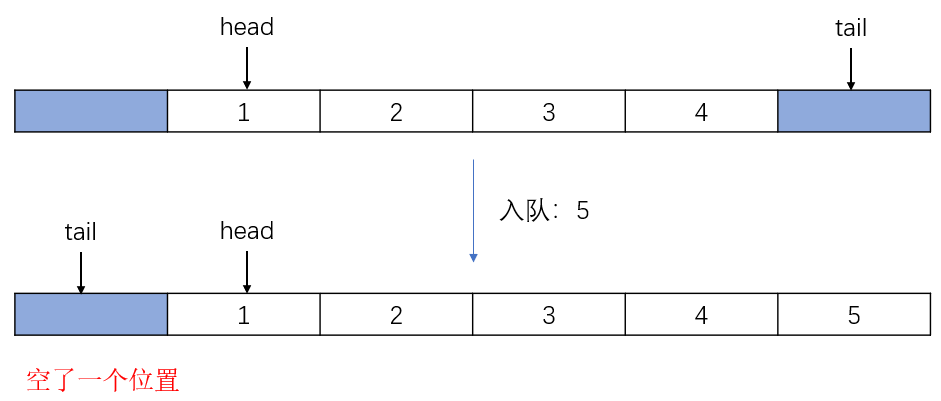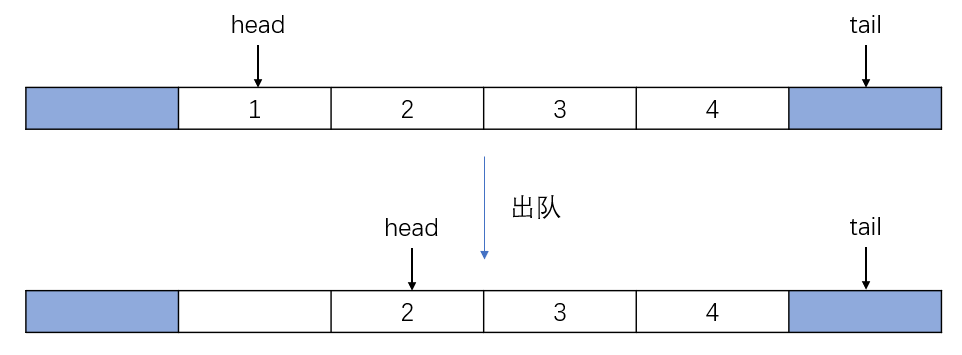head == (tail + 1) % size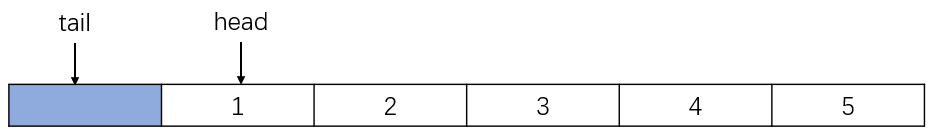### 4.1、基本函数实现

public int getCapacity() {  //判断容器是否为空
return data.length - 1;
}

@Override
public int getSize() {  //获取队列实际数据含量
return size;
}

@Override
public boolean isEmpty() {  //当头和尾重合即为空
}


### 4.2、进出队列函数

@Override
public void enqueue(E e) {
if ((tail + 1) % data.length == head)  // 判断数组中是否已经存满
resize(getCapacity() * 2);

data[tail] = e;
tail = (tail + 1) % data.length;  //tail向后自增
size++;  // 维护size
}

@Override
public E dequeue() {
if (isEmpty()) {
throw new IllegalArgumentException("Cannot dequeue from an empty queue");
}
size--;  // 维护size
if (size == getCapacity() / 4 && getCapacity() / 2 != 0) {  // 防止时间复杂度震荡并保持整除
resize(getCapacity() / 2);
}
return temp;
}


private void resize(int newCapacity) {
E[] newData = (E[]) new Object[newCapacity + 1];
for (int i = 0; i < size; i++)
newData[i] = data[(i + head) % data.length];
tail = size;
data = newData;
}


### 4.3、查询操作

@Override
public E getFront() {
if (isEmpty()) {
throw new IllegalArgumentException("Cannot getFront from an empty queue");
}
}


## 最后